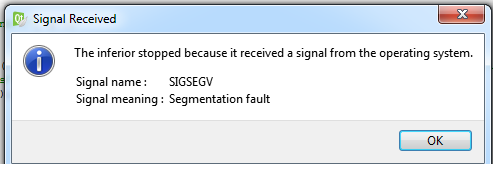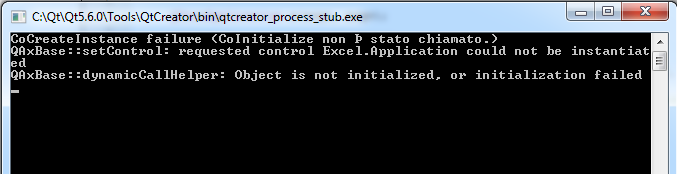# QAxObject and Excel

• Hi for all.
I am trying this code but it does not work. I get a crash at the execution of the instruction
--->:QAxObject* workbook = workbooks->querySubObject( "Open(const QString&)", "C:\myTest\1.xls" );The code seems correct. Other examples are made like this. The xls file is also in the correct folder but it does not work

``````#include <QCoreApplication>
#include <QAxObject>

int main(int argc, char *argv[])
{

QCoreApplication a(argc, argv);

QAxObject* excel = new QAxObject( "Excel.Application", 0 );
QAxObject* workbooks = excel->querySubObject( "Workbooks" );
QAxObject* workbook = workbooks->querySubObject( "Open(const QString&)", "C:\\myTest\\1.xls" );
QAxObject* sheets = workbook->querySubObject( "Worksheets" );
QList<QVariantList> data; //Data list from excel, each QVariantList is worksheet row

//worksheets count
int count = sheets->dynamicCall("Count()").toInt();

count = sheets->property("Count").toInt();
for (int i=1; i<=count; i++) //cycle through sheets
{
//sheet pointer
QAxObject* sheet = sheets->querySubObject( "Item( int )", i );

QAxObject* rows = sheet->querySubObject( "Rows" );
int rowCount = rows->dynamicCall( "Count()" ).toInt(); //unfortunately, always returns 255, so you have to check somehow validity of cell values
QAxObject* columns = sheet->querySubObject( "Columns" );
int columnCount = columns->property("Count").toInt();
for (int row=1; row <= rowCount; row++)
{
QVariantList dataRow;
bool isEmpty = true; //when all the cells of row are empty, it means that file is at end (of course, it maybe not right for different excel files. it's just criteria to calculate somehow row count for my file)
for (int column=1; column <= columnCount; column++)
{
//Do something usefule here
}
if (isEmpty) //criteria to get out of cycle
break;
data.append(dataRow);

}
}

workbook->dynamicCall("Close()");
excel->dynamicCall("Quit()");
return a.exec();
}

``````

• Hi, QAxObject does not work well in a without a message pump to handle the COM events, so instead of a console application, you could put your code inside a Qt Widgets app.
So create a new, vanilla Widgets app, add axcontainer to the QT += line in the .pro file and edit the mainwindow.cpp:

``````ui->setupUi(this);
// inside MainWindow's ctor, add this:

auto excel     = new QAxObject("Excel.Application");
auto workbooks = excel->querySubObject("Workbooks");
auto workbook  = workbooks->querySubObject("Open(const QString&)","C:\\myTest\\1.xls");
auto sheets    = workbook->querySubObject("Worksheets");

QList<QVariantList> data; //Data list from excel, each QVariantList is worksheet row

//worksheets count
int count = sheets->dynamicCall("Count()").toInt();

count = sheets->property("Count").toInt();
for (int i=1; i<=count; i++) //cycle through sheets
{
//sheet pointer
QAxObject* sheet = sheets->querySubObject( "Item( int )", i );

QAxObject* rows = sheet->querySubObject( "Rows" );
int rowCount = rows->dynamicCall( "Count()" ).toInt(); //unfortunately, always returns 255, so you have to check somehow validity of cell values
QAxObject* columns = sheet->querySubObject( "Columns" );
int columnCount = columns->property("Count").toInt();
for (int row=1; row <= rowCount; row++)
{
QVariantList dataRow;
bool isEmpty = true; //when all the cells of row are empty, it means that file is at end (of course, it maybe not right for different excel files. it's just criteria to calculate somehow row count for my file)
for (int column=1; column <= columnCount; column++)
{
qDebug() << row << column;
//Do something usefule here
}
if (isEmpty) //criteria to get out of cycle
break;
data.append(dataRow);
}
}

workbook->dynamicCall("Close()");
excel->dynamicCall("Quit()");
}``````

• Hi again, correction to my previous post:

QAxObject works without a message pump and works in a console app. You only have to change your QCoreApplication to QApplication.

Change these 2 lines in your example:

``````#include <QApplication>      //here
#include <QAxObject>

int main(int argc, char *argv[])
{
QApplication a(argc, argv);    // and here

QAxObject* excel = new QAxObject( "Excel.Application", 0 );

...
``````

• @newbieQTDev You should always check the pointers before you use them! So, check whether workbook is a valid pointer before dereferencing it.

• Hi hskoglund.
I changed the code how you said but i got the same error.

• Hmm, I did a small test Qt console app with your code (with the changes: #include <QApplication> and QApplication a(argc, argv);
and an Excel test file and it worked fine. When I insert qDebug() << row << column in the inner loop it printed all the columns and rows.

Oh, there's one more reason for the error to occur: if you are running your Qt program on a PC without any Excel installed.

• @hskoglund Hello, I have used your code to read an excel file by using QAxobject and it worked perfect, but in my case, the chart has many merged cells. I need to know the number of rows which a merged cell occupies. What should I do? Thank you so much.

• @Arash_Vsh
Qt `QAxObject` just lets you interact with ActiveX/Excel etc. It does not document what you can do in them. You must read Excel VBA documentation/examples for any questions like this, e.g. start from https://docs.microsoft.com/en-us/office/vba/api/overview/excel/object-model

• If you want to read any excel file then use `QSqlDatabase` For It

my personal experience saying that `QAxObject` take Lot's of time for read any kind of Excel file
And `QAxObject` Very complicated Concept

you can see my code for Read Any Kind Of Excel File

``````QSqlDatabase db = QSqlDatabase::addDatabase("QODBC", "xlsx_connection");
db.setDatabaseName("DRIVER={Microsoft Excel Driver (*.xls, *.xlsx, *.xlsm, *.xlsb)};DBQ=" + QString(FilePath) + "");
if(db.open())
qDebug() << "Excel Is Connected";
else
qDebug() << "Excel Is Not Connected";

QSqlQuery query(db);
query.prepare("SELECT * FROM [" + QString("Sheet1") + "\$]"); // Select range, place A1:B5 after \$
if(query.exec())
{
while (query.next())
{
}
}
``````

for this You must have to Install Mysql Driver For Excel

• @Ketan__Patel__0011
If one does it this way, the issue then is where do you find details on what tables/columns/links/operations etc. can you perform?

I can see one might start by exploring down from https://docs.microsoft.com/en-us/sql/odbc/microsoft/microsoft-excel-driver-programming-considerations . Does that sound right, or do you have a better resource for what/how you can do to Excel across SQL/ODBC?

• @JonB

Yes i can perform all are the operation like select Particular Columns or etc..

In My case i am using this Solution And it give me complate solution

You can see

query.prepare("SELECT * FROM [" + QString("Sheet1") + "\$]"); // Select range, place A1:B5 after \$

Here I am using "Sheet1" As My table and i am fatching All Columns data of My Table
So You can simply Get the Data from pass columns index like this "Follow My code"

``````    if(query.exec())
{
while (query.next())
{
QString column1= query.value(0).toString();
QString column2 = query.value(1).toString();
QString column3 = query.value(2).toString();
QString column4 = query.value(3).toString();
}
}
``````

• @Ketan__Patel__0011
Yes indeed, for an example of "select some adjacent columns and fetch their values".

But have a look at, say, https://docs.microsoft.com/en-us/sql/odbc/microsoft/sqlgetinfo-returned-values-for-excel?view=sql-server-ver15. Or, how do you set up an Excel Pivot table? What about the manipulations available of all the various object types described in the VBA Excel reference? That sort of thing is what I was thinking one needs documentation for.

• @JonB

I have not work with Some Advanced level.
So I can't explain much more for it.

but you can use this concept for read simple excel file and fatch the data from your excel sheet table.

And I Will Start the work on Excel Pivot table and other Advanced concepts.
Then i will drop the solution.

• @Ketan__Patel__0011
Hi Ketan.
No need for you to actually find solutions to what I wrote, they are just examples of what a user might want. Your suggestion of using SQL/ODBC instead of ActiveX is interesting. All I was saying is that if the OP wishes to go down that route they might use https://docs.microsoft.com/en-us/sql/odbc/microsoft/microsoft-excel-driver-programming-considerations as a starting point for documentation. That was all.

• @JonB

I Didn't get the any message from User(newbieQTDev ) so he Actually want Solution for Excel file Reading using ActiveX Then i Will Drop the solution for it.

I have both way solution for it But `SQL/ODBC` Nice And easy way for Excel file reading.

And you are Agree with my Concept then Mark My Post As The Correct Answer.

• Actually want Solution for Excel file Reading using ActiveX Then i Will Drop the solution for it

Even if he does want only ActiveX, do not drop your SQL/ODBC solution here! It is a very useful alternative way of going about things, depending on what is wanted. Even if it is not right for this user, it is useful to read for others coming to this topic :)

• @JonB

So Should I Delete My Post ?

• @Ketan__Patel__0011
Noooo!!! Not at all, I am saying your post is very useful. Maybe not to this OP, but potentially anyway to others. It is a good post :)

• hi
@Ketan__Patel__0011 said in QAxObject and Excel:

So Should I Delete My Post ?

No, don't delete, i belive the OP is able to chose the right solution for what he needs

• @LeLev

Okay i will Restore The Post

Sorry To Say But What Is Meaning Of

@LeLev said in QAxObject and Excel:

OP
?

• @Ketan__Patel__0011

OP == "Original Poster", the person who starts a thread.

• @Ketan__Patel__0011 the person who created this thread, (Original Poster )

• @LeLev

Okay i got it Thank You

• @JonB

I Restored it

• @Ketan__Patel__0011 I agree with you. AxObject is very slow especially in Save Data.

• @Morteza_Mahmoudi

Happy To Help